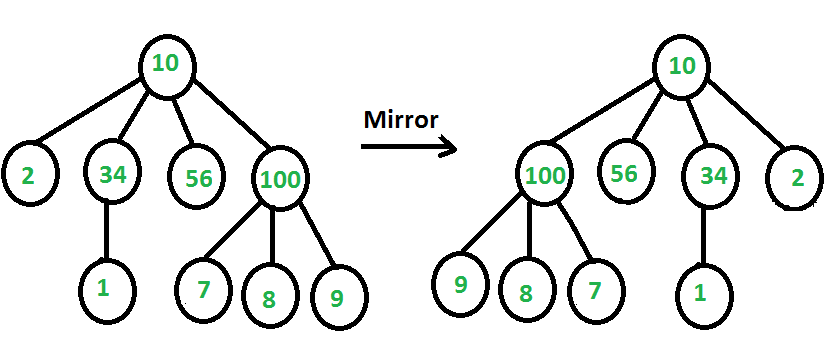# Mirror of n-ary Tree

• Difficulty Level : Medium
• Last Updated : 20 Jan, 2022

Given a Tree where every node contains variable number of children, convert the tree to its mirror. Below diagram shows an example.We strongly recommend you to minimize your browser and try this yourself first.
Node of tree is represented as a key and a variable sized array of children pointers. The idea is similar to mirror of Binary Tree. For every node, we first recur for all of its children and then reverse array of children pointers. We can also do these steps in other way, i.e., reverse array of children pointers first and then recur for children.
Below is C++ implementation of above idea.

## C++

 `// C++ program to mirror an n-ary tree` `#include ` `using` `namespace` `std;`   `// Represents a node of an n-ary tree` `struct` `Node` `{` `    ``int` `key;` `    ``vectorchild;` `};`   `// Function to convert a tree to its mirror` `void` `mirrorTree(Node * root)` `{` `    ``// Base case: Nothing to do if root is NULL` `    ``if` `(root==NULL)` `        ``return``;`   `    ``// Number of children of root` `    ``int` `n = root->child.size();`   `    ``// If number of child is less than 2 i.e.` `    ``// 0 or 1 we do not need to do anything` `    ``if` `(n < 2)` `        ``return``;`   `    ``// Calling mirror function for each child` `    ``for` `(``int` `i=0; ichild[i]);`   `    ``// Reverse vector (variable sized array) of child` `    ``// pointers` `    ``reverse(root->child.begin(), root->child.end());` `}`   `// Utility function to create a new tree node` `Node *newNode(``int` `key)` `{` `    ``Node *temp = ``new` `Node;` `    ``temp->key = key;` `    ``return` `temp;` `}`   `// Prints the n-ary tree level wise` `void` `printNodeLevelWise(Node * root)` `{` `    ``if` `(root==NULL)` `        ``return``;`   `    ``// Create a queue and enqueue root to it` `    ``queueq;` `    ``q.push(root);`   `    ``// Do level order traversal. Two loops are used` `    ``// to make sure that different levels are printed` `    ``// in different lines` `    ``while` `(!q.empty())` `    ``{` `        ``int` `n = q.size();` `        ``while` `(n>0)` `        ``{` `            ``// Dequeue an item from queue and print it` `            ``Node * p = q.front();` `            ``q.pop();` `            ``cout << p->key << ``" "``;`   `            ``// Enqueue all childrent of the dequeued item` `            ``for` `(``int` `i=0; ichild.size(); i++)` `                ``q.push(p->child[i]);` `            ``n--;` `        ``}`   `        ``cout << endl; ``// Separator between levels` `    ``}` `}`   `// Driver program` `int` `main()` `{` `    ``/*   Let us create below tree` `    ``*              10` `    ``*        /   /    \   \` `    ``*        2  34    56   100` `    ``*                 |   /  | \` `    ``*                 1   7  8  9` `    ``*/` `    ``Node *root = newNode(10);` `    ``(root->child).push_back(newNode(2));` `    ``(root->child).push_back(newNode(34));` `    ``(root->child).push_back(newNode(56));` `    ``(root->child).push_back(newNode(100));` `    ``(root->child->child).push_back(newNode(1));` `    ``(root->child->child).push_back(newNode(7));` `    ``(root->child->child).push_back(newNode(8));` `    ``(root->child->child).push_back(newNode(9));`   `    ``cout << ``"Level order traversal Before Mirroring\n"``;` `    ``printNodeLevelWise(root);`   `    ``mirrorTree(root);`   `    ``cout << ``"\nLevel order traversal After Mirroring\n"``;` `    ``printNodeLevelWise(root);`   `    ``return` `0;` `}`

## Python3

 `# Python program to mirror an n-ary tree`   `# Represents a node of an n-ary tree` `class` `Node :`   `    ``# Utility function to create a new tree node` `    ``def` `__init__(``self` `,key):` `        ``self``.key ``=` `key ` `        ``self``.child ``=` `[]`     `# Function to convert a tree to its mirror` `def` `mirrorTree(root):` `    `  `    ``# Base Case : nothing to do if root is None` `    ``if` `root ``is` `None``:` `        ``return` `    `  `    ``# Number of children of root ` `    ``n ``=` `len``(root.child)`   `    ``# If number of child is less than 2 i.e. ` `    ``# 0 or 1 we don't need to do anything` `    ``if` `n <``2` `:` `        ``return` `    `  `    ``# Calling mirror function for each child` `    ``for` `i ``in` `range``(n):` `        ``mirrorTree(root.child[i]);` `    `  `    ``# Reverse variable sized array of child pointers` `    ``root.child.reverse()` `    `    `# Prints the n-ary tree level wise`   `def` `printNodeLevelWise(root):` `    ``if` `root ``is` `None``:` `        ``return` `    `  `    ``# create a queue and enqueue root to it` `    ``queue ``=` `[]` `    ``queue.append(root)`   `    ``# Do level order traversal. Two loops are used` `    ``# to make sure that different levels are printed` `    ``# in different lines` `    ``while``(``len``(queue) >``0``):`   `        ``n ``=` `len``(queue)` `        ``while``(n > ``0``) :`   `            ``# Dequeue an item from queue and print it` `            ``p ``=` `queue[``0``]` `            ``queue.pop(``0``)` `            ``print``(p.key,end``=``" "``)` `    `  `            ``# Enqueue all children of the dequeued item` `            ``for` `index, value ``in` `enumerate``(p.child):` `                ``queue.append(value)`   `            ``n ``-``=` `1` `        ``print``() ``# Separator between levels` `        `    `# Driver Program`   `    ``"""   Let us create below tree` `    ``*              10` `    ``*        /   /    \   \` `    ``*        2  34    56   100` `    ``*                 |   /  | \` `    ``*                 1   7  8  9` `    ``"""`   `root ``=` `Node(``10``)` `root.child.append(Node(``2``))` `root.child.append(Node(``34``))` `root.child.append(Node(``56``))` `root.child.append(Node(``100``))` `root.child[``2``].child.append(Node(``1``))` `root.child[``3``].child.append(Node(``7``))` `root.child[``3``].child.append(Node(``8``))` `root.child[``3``].child.append(Node(``9``))`   `print` `(``"Level order traversal Before Mirroring"``)` `printNodeLevelWise(root)`   `mirrorTree(root)` `    `  `print` `(``"\nLevel Order traversal After Mirroring"``)` `printNodeLevelWise(root)`

## Javascript

 ``

Output:

```Level order traversal Before Mirroring
10
2 34 56 100
1 7 8 9

Level order traversal After Mirroring
10
100 56 34 2
9 8 7 1 ```

Thanks to Nitin Agrawal for providing initial implementation. Please write comments if you find anything incorrect, or you want to share more information about the topic discussed above

My Personal Notes arrow_drop_up
Recommended Articles
Page :